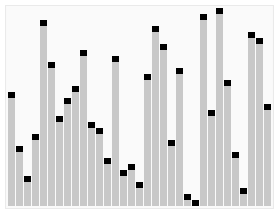# 交换排序

```#include<iostream>
using namespace std;
void bubbleSort(int *a, int n);
void bubbleSort(int *a, int n) {
int i, j, temp;
for (j = 0; j < n - 1; j++)
for (i = 0; i < n - 1 - j; i++)
{
if (a[i] > a[i + 1])
{
temp = a[i];
a[i] = a[i + 1];
a[i + 1] = temp;
}
}
}
int main() {
int arry[] = { 11,5,2,1,10,4,6,8,7 };
int length = sizeof(arry) / sizeof(int);
bubbleSort(arry, length);
for (int i = 0; i <length; i++) {
cout << arry[i] << " ";
}
cout << endl;
}```

1.从数列中挑出一个元素，称为"基准"（pivot）
2.重新排序数列，所有元素比基准值小的摆放在基准前面，所有元素比基准值大的摆在基准的后面（相同的数可以到任一边）。在这个分区结束之后，该基准就处于数列的中间位置。这个称为分区（partition）操作。
3.递归地（recursive）把小于基准值元素的子数列和大于基准值元素的子数列排序。```#include<iostream>
using namespace std;
void quickSort(int *arry,int,int length);
void quickSort(int *arry, int low, int high) {

if (low >= high) {
return;
}
int flag = arry[low];//取数组的第一个元素作为"轴值"
int last = high;  //这里注意，需要对函数常数参数进行保存，在递归中需要用到。在进行第一次排序后，low和high的值都改变且相等。
int first = low;
while (low < high) {

while (low < high&&arry[high] >=flag) {

--high;
}
arry[low] = arry[high];```
``` while (low < high&&arry[low] <= flag) {

++low;
}
arry[high] = arry[low];
}
arry[low] = flag;//第一次排序后，把flag赋值给"轴值"
quickSort(arry, first,low-1);//递归
quickSort(arry, low + 1, last);
}
int main(){
int arry[] = {23,13,49,6,31,19,28};
int length = sizeof(arry) / sizeof(int);
quickSort(arry,0,length-1);
for (int i = 0; i < length; i++) {
cout << arry[i] << " ";
}
cout << endl;
} ```

• 博文量
159
• 访问量
982620